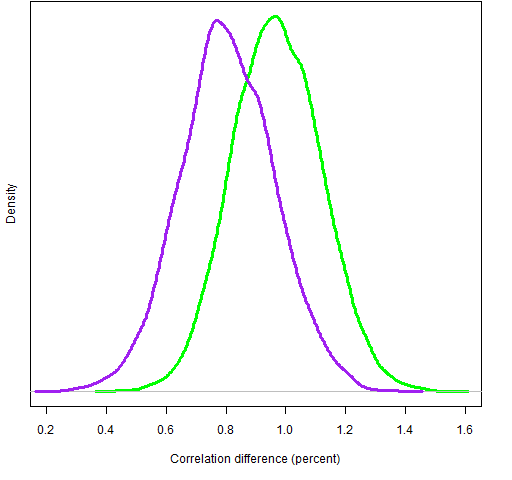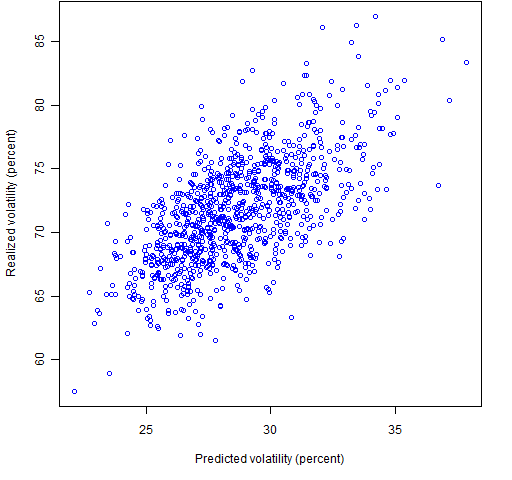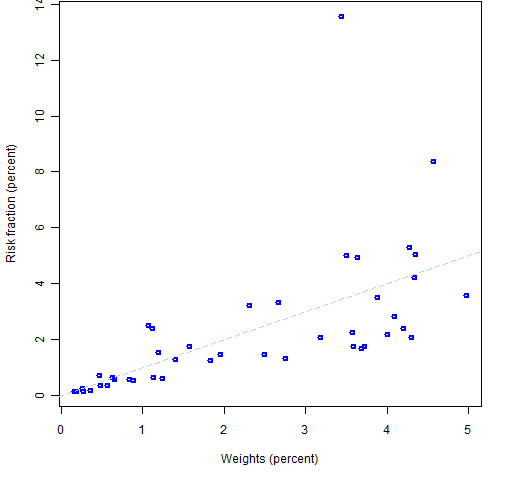# Posts Tagged ‘ risk fraction ’

## 2 dimensions of portfolio diversity

July 16, 2012
ByPortfolio diversity is a balancing act. Previously The post “Portfolio diversity” talked about the role of the correlation between assets and the portfolio.  The current post fills a hole in that post. The 2 dimensions asset-portfolio correlation Each asset in the universe has a correlation with the portfolio.  If there are any assets that have … Continue reading →

## Specific differences between Ledoit-Wolf and factor models

May 22, 2011
ByWhat can we learn about the difference in structure between a Ledoit-Wolf variance matrix and a corresponding factor model variance? Previously We’ve generated a set of random portfolios with constraints on the risk fractions of a Ledoit-Wolf variance matrix, and a corresponding set of random portfolios with risk fraction constraints from a statistical factor model. … Continue reading →

## Again with Ledoit-Wolf and factor models

May 4, 2011
ByWe come closer to a definitive answer on the relative merit of Ledoit-Wolf shrinkage versus a statistical factor model for variance matrices. Previously This post builds on the post entitled: A test of Ledoit-Wolf versus a factor model That post depended on some posts previous to it. New information Previously we generated random portfolios with … Continue reading →

## A test of Ledoit-Wolf versus a factor model

April 27, 2011
ByStatistical factor models and Ledoit-Wolf shrinkage are competing methods for estimating variance matrices of returns.  So which is better?  This adds a data point for answering that question. Previously There are past blog posts on: the idea of variance matrices factor models of variance The data in this post are from the blog posts: “Weight … Continue reading →

## Risk fraction constraints and volatility

April 21, 2011
ByWhat is the effect on predicted and realized volatility of substituting risk fraction constraints for weight constraints? Previously This post depends on two previous blog posts: “Unproxying weight constraints” “Weight compared to risk fraction” The exact same sets of random portfolios are used in this post that were generated in the second of these. Payoff … Continue reading →

## Weight compared to risk fraction

April 18, 2011
ByHow well do asset weight constraints constrain risk? The setup In “Unproxying weight constraints” I claimed that many constraints on asset weights are really a proxy for constraining risk. That is not a problem if weights are a good proxy for risk.  So the question is: how good of a proxy are they? To give … Continue reading →